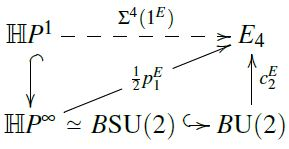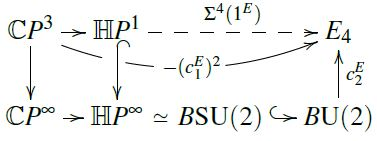# Start a new discussion

## Not signed in

Want to take part in these discussions? Sign in if you have an account, or apply for one below

## Site Tag Cloud

Vanilla 1.1.10 is a product of Lussumo. More Information: Documentation, Community Support.

• CommentRowNumber1.
• CommentAuthorUrs
• CommentTimeNov 25th 2020
• (edited Dec 31st 2020)

starting something. For the moment just checking what literature exists. So far I am aware of this:

• CommentRowNumber2.
• CommentAuthorDavid_Corfield
• CommentTimeNov 25th 2020

Just seeing what it is, I came across this PhD thesis by Craig Matthew Laughton, Quasitoric manifolds and cobordism theory. A definition at Example 2.2.5.

• CommentRowNumber3.
• CommentAuthorUrs
• CommentTimeNov 25th 2020

Thanks! I have added this as Laughton 08. Also at MSp.

• CommentRowNumber4.
• CommentAuthorUrs
• CommentTimeNov 25th 2020

added the obstruction-theoretic argument for the existence of quaternionic orientations (here) together with the conclusion that $KO$ is quaternionic orientable (here)

• CommentRowNumber5.
• CommentAuthorDavid_Corfield
• CommentTimeNov 25th 2020

There’s also the example

Among quaternionic oriented cohomology theories, quaternionic cobordism is universal in the analogous fashion to complex cobordism (Laughton, Example 2.2.9)

• CommentRowNumber6.
• CommentAuthorDavid_Corfield
• CommentTimeNov 25th 2020

I’ll add that, but it should be said more precisely.

• CommentRowNumber7.
• CommentAuthorUrs
• CommentTimeNov 25th 2020
• (edited Nov 25th 2020)

I made quaternionic cobordism redirect to MSp

• CommentRowNumber8.
• CommentAuthorUrs
• CommentTimeDec 31st 2020
• (edited Dec 31st 2020)

Implicit (?) in Baker 92 is the suggestion that we get a quaternionic orientation $\tfrac{1}{2}p_1^E$ from the second Conner-Floyd Chern class $c_2^E$ of any complex orientation $c_1^E$, by defining it via the solid triangle here:It remains to see that this really satisfies the defining condition, hence that the dashed morphism makes the top triangle homotopy-commute.

To that end I am looking at the following solid diagram:That makes it rather plausible. But I am still looking for a formal way to prove it.

• CommentRowNumber9.
• CommentAuthorDylan Wilson
• CommentTimeDec 31st 2020
@Urs, (c_1^E)^2 is zero when restricted to CP^2, so it factors through CP^3/CP^2 = HP^1 as indicated
• CommentRowNumber10.
• CommentAuthorUrs
• CommentTimeDec 31st 2020
• (edited Jan 21st 2021)

Thanks, Dylan! I had just seen it myself and was typing it up:

[edit on 21st Jan 2021: image removed]

[edit on 21st Jan 2021: Hm, no, this argument is wrong. For instance, it would imply that all degree-6 cohomology of $\mathbb{C}P^3$ comes pulled back from $S^4$, which is nonsense. I guess the problem is that the square (b) is not actually a homotopy pushout. It seems to be from the pasting law applied to its pasting with (c), but that needs an argument that the homotopies filling these squares match up, and that might fail.]

• CommentRowNumber11.
• CommentAuthorUrs
• CommentTimeDec 31st 2020
• (edited Jan 21st 2021)

[removed]

• CommentRowNumber12.
• CommentAuthorUrs
• CommentTimeDec 31st 2020

Next, I’d like to refine this statement to finite-stage orientations:

It ought to be true that a quaternionic orientation “at stage $n$”, i.e. an extension of this form:

$\array{ \mathbb{H}P^1 &\overset{\Sigma^4(1^E)}{\longrightarrow}& E_4 \\ \big\downarrow & \nearrow_{\mathrlap{ \tfrac{1}{2}p_1^E }} \\ \mathbb{H}P^n }$

may analogously be induced from a complex orientation at stage $2n + 1$, i.e. from an extension of this form

$\array{ \mathbb{C}P^1 &\overset{\Sigma^2(1^E)}{\longrightarrow}& E_2 \\ \big\downarrow & \nearrow_{\mathrlap{ c_1^E }} \\ \mathbb{C}P^{2n+1} }$

By adapting the above proof and using the construction of the Conner-Floyd classes here, this should be implied from observing that the canonical map

$\mathbb{C}P^{2n+1} \longrightarrow \mathbb{H}P^n$

is in fact the complex projective bundle of the canonical quaternionic line bundle on $\mathbb{H}P^n$.

This ought to be true (e.g. the fiber is $\mathbb{C}P^1$, as it should be for the projective bundle of a rank-2 complex vector bundle), but I still need to write a formal proof.

• CommentRowNumber13.
• CommentAuthorUrs
• CommentTimeJan 3rd 2021

• CommentRowNumber14.
• CommentAuthorUrs
• CommentTimeJan 24th 2021
• (edited Jan 24th 2021)

The argument in #9, #10 above was not correct (for instance: the same argument would immediately imply that $\mathbb{C}P^3$ has no ordinary cohomolgy in degree 6…).

The following argument should work. It’s essentially a standard basic argument in complex oriented cohomology theory, and I suppose I am making a fool of myself here in going on about this at all. But here goes:

To recall, the question was:

How to formally show that every complex orientation $c^E_1$ induces a quaternionic orientation by setting $\tfrac{1}{2}p^E_1 \;\coloneqq\; c^E_2$?

First, a quick abstract way to see it:

Just as complex orientations are co-represented by the ring spectrum $MU$ (all homotopy-commutative), so quaternionic orientations are co-represented by the ring spectrum $MSp$ (by the same proof, just with the evident substitutions), and the evident map $MSp \longrightarrow MU$ is a ring spectrum homomorphisms. This way complex orientations $MU \to E$ immediately induce quaternionic representations $MSp \to E$ by precomposition with $MSp \to MU$.

Unravelling this, what is happening under the hood:

1. Under the equivalence $\mathbb{H}P^\infty \simeq Th\big( \mathcal{L}^\ast_{\mathbb{H}P^\infty} \big)$ the following two inclusions are equivalent (by this diagram):

1. the canonical inclusion $S^4 \simeq \mathbb{H}P^1 \hookrightarrow \mathbb{H}P^\infty$;

2. the canonical inclusion $S^4 \simeq Th\big( \mathbb{H} \big) \hookrightarrow Th\big( \mathcal{L}^\ast_{\mathbb{H}P^\infty} \big)$.

2. The fact that the Conner-Floyd Chern class $c^E_2$ is a Thom class for complex rank-2 bundles says it restricts to unity along the second of these maps.

But by the equivalence to the first of these maps, it also restricts to unity there, and this was to be shown.

Of course the very same argument run in reverse shows that $c^E_1$ itself really gives an orientation of complex line bundles. So, nothing to see here, please move along.